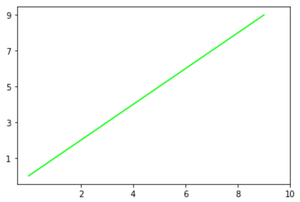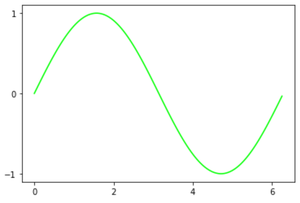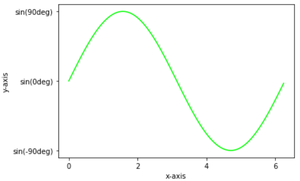Open in App
Not now

# Matplotlib – Setting Ticks and Tick Labels

• Difficulty Level : Expert
• Last Updated : 26 Dec, 2020

In this article, we are going to discuss how set Ticks and Tick labels in a graph.

Ticks are the markers denoting data points on the axes and tick labels are the name given to ticks. By default matplotlib itself marks the data points on the axes but it has also provided us with setting their own axes having ticks and tick labels of their choice.

Methods used:

• plt.axes(*args, emit=True, **kwargs): For setting the axes for our plot with parameter rect as [left,bottom,width,height] for  setting axes position.
• plt.axes().set_xticks() and plt.axes().set_yticks() : For setting ticks on x-axis and y-axis respectively. having data in form of a list set as parameter.
• plt.axes().set_xlabels() and plt.axes().set_ylabels() : To set labels of our ticks with parameters in form of list.

Below are some examples which depict how to add ticks and ticklabels in a plot:

Example 1:

## Python3

 `# import required module``import` `matplotlib.pyplot as plt`` ` `# assign coordinates``x ``=` `y ``=` `[i ``for` `i ``in` `range``(``0``, ``10``)]``ax ``=` `plt.axes()`` ` `# depict illustration``plt.plot(x, y, color``=``"lime"``)`` ` `# setting ticks for x-axis``ax.set_xticks([``2``, ``4``, ``6``, ``8``, ``10``])`` ` `# setting ticks for y-axis``ax.set_yticks([``1``, ``3``, ``5``, ``7``, ``9``])`` ` `plt.show()`

Output:Example 2:

## Python3

 `# import required module``import` `matplotlib.pyplot as plt`` ` `# assign coordinates``x ``=` `y ``=` `[i ``for` `i ``in` `range``(``0``, ``10``)]``ax ``=` `plt.axes()`` ` `# depict illustration``plt.plot(x, y, color``=``"lime"``)`` ` `# setting ticks for x-axis``ax.set_xticks([``2``, ``4``, ``6``, ``8``, ``10``])`` ` `# setting label for x tick``ax.set_xticklabels([``'Geeks'``, ``'for'``, ``'geeks'``, ``'!'``])`` ` `# setting ticks for y-axis``ax.set_yticks([``1``, ``3``, ``5``, ``7``, ``9``])`` ` `# setting label for y tick``ax.set_yticklabels([``'A'``, ``'B'``, ``'C'``, ``'D'``])`` ` `plt.show()`

Output:Example 3:

## Python3

 `# import required modules``import` `matplotlib.pyplot as plt``import` `numpy as np``import` `math`` ` `# assign coordinates``x ``=` `np.arange(``0``, math.pi``*``2``, ``0.05``)``y ``=` `np.sin(x)``ax ``=` `plt.axes()`` ` `# depict illustration``plt.plot(x, y, color``=``"lime"``)`` ` `# setting ticks for x-axis``ax.set_xticks([``0``, ``2``, ``4``, ``6``])`` ` `# setting ticks for y-axis``ax.set_yticks([``-``1``, ``0``, ``1``])`` ` `plt.show()`

Output:Example 4:

## Python3

 `# import required modules``import` `matplotlib.pyplot as plt``import` `numpy as np``import` `math`` ` `# assign coordinates``x ``=` `np.arange(``0``, math.pi``*``2``, ``0.05``)``y ``=` `np.sin(x)``ax ``=` `plt.axes()``plt.xlabel(``"x-axis"``)``plt.ylabel(``"y-axis"``)`` ` `# depict illustration``plt.plot(x, y, color``=``"lime"``)`` ` `# setting ticks for x-axis``ax.set_xticks([``0``, ``2``, ``4``, ``6``])`` ` `# setting ticks for y-axis``ax.set_yticks([``-``1``, ``0``, ``1``])`` ` `# setting label for y tick``ax.set_yticklabels([``"sin(-90deg)"``, ``"sin(0deg)"``, ``"sin(90deg)"``])`` ` `plt.show()`

Output:My Personal Notes arrow_drop_up